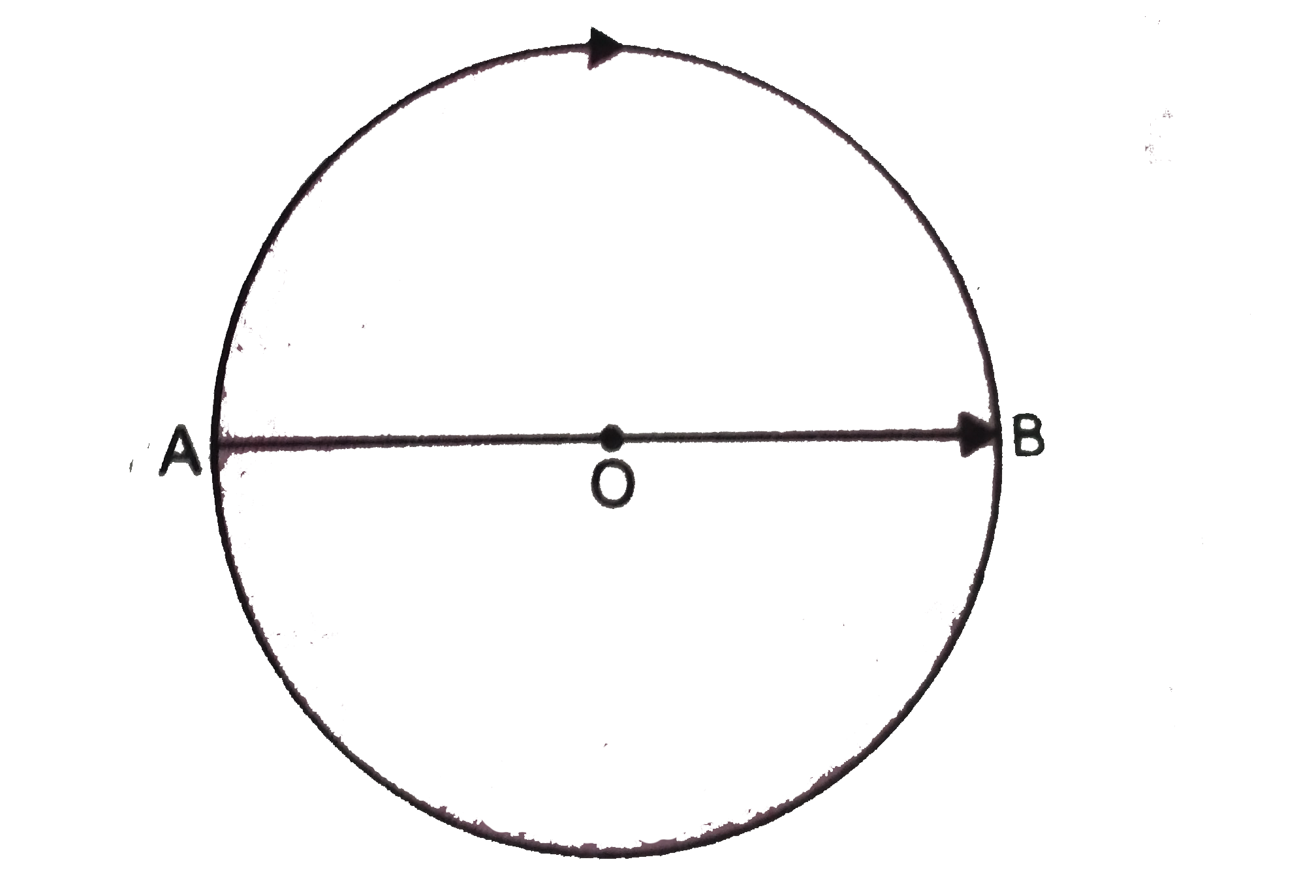Doubtnut App - Get 50 Lakh+ video solutions better than GoogleInstall Now

# An athlete complete one round of a circular track of diameter 200m in 40s. What will be the distance covered and the displacement at the end of 2 minutes 20s ?

Text Solution
Verified by Experts

## As shown in Figure, diametre of circular track, AB=200m. Time taken to complete one round =40s Total time =2min20s=(2×60+20)s=140s Number of rounds completed =14040=3.5 Distance covered =2πr×3.5 =2×(22/7)×(200/2)×3.5m=2200m If the athlete starts from A, on completion of 3.5 rounds, he will be at B. Thus, displacement =−−→AB=200m (in magnitude).